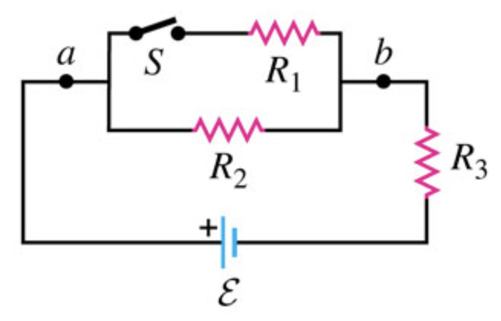# Problem: In the circuit shown below, ε = 71.0 V, R1 = 4.00 Ω, R2 = 6.00 Ω, R3 = 3.00 Ω.A) What is the potential difference Vab between points a and b when the switch S is open?B) What is the potential difference Vab between points a and b when the switch S is closed?C) For the 4.00 Ω resistor, calculate the current through the resistor with S open.D) For the 4.00 Ω resistor, calculate the current through the resistor with S closed.E) For the 4.00 Ω resistor, calculate the current through the resistor with S closed.F) For the 6.00 Ω resistor, calculate the current through the resistor with S closed.G) For the 3.00 Ω resistor, calculate the current through the resistor with S open.H) For the 3.00 Ω resistor, calculate the current through the resistor with S closed.I) For each resistor, does the current increase or decrease when S is closed?

###### FREE Expert Solution

Equivalent resistance for resistors in parallel:

$\overline{)\frac{\mathbf{1}}{{\mathbf{R}}_{\mathbf{eq}}}{\mathbf{=}}\frac{\mathbf{1}}{{\mathbf{R}}_{\mathbf{1}}}{\mathbf{+}}\frac{\mathbf{1}}{{\mathbf{R}}_{\mathbf{2}}}{\mathbf{+}}{\mathbf{.}}{\mathbf{.}}{\mathbf{.}}{\mathbf{+}}\frac{\mathbf{1}}{{\mathbf{R}}_{\mathbf{n}}}}$

or for 2 resistors:

$\overline{){{\mathbf{R}}}_{{\mathbf{eq}}}{\mathbf{=}}\frac{{\mathbf{R}}_{\mathbf{1}}{\mathbf{R}}_{\mathbf{2}}}{{\mathbf{R}}_{\mathbf{1}}\mathbf{+}{\mathbf{R}}_{\mathbf{2}}}}$

Equivalent resistance for resistors in series:

$\overline{){{\mathbf{R}}}_{{\mathbf{eq}}}{\mathbf{=}}{{\mathbf{R}}}_{{\mathbf{1}}}{\mathbf{+}}{{\mathbf{R}}}_{{\mathbf{2}}}{\mathbf{+}}{\mathbf{.}}{\mathbf{.}}{\mathbf{.}}{\mathbf{+}}{{\mathbf{R}}}_{{\mathbf{n}}}}$

Ohm's law:

$\overline{){\mathbit{V}}{\mathbf{=}}{\mathbit{i}}{\mathbit{R}}}$

A)

When switch  S is open R2 and R3 are connected in series.

Req = R2 + R3 = 6.00 + 3.00 = 9.00Ω

i = ε/Req = 71.0/9.00 = 7.89 A

93% (345 ratings)###### Problem Details

In the circuit shown below, ε = 71.0 V, R1 = 4.00 Ω, R2 = 6.00 Ω, R3 = 3.00 Ω.A) What is the potential difference Vab between points a and b when the switch S is open?

B) What is the potential difference Vab between points a and b when the switch S is closed?

C) For the 4.00 Ω resistor, calculate the current through the resistor with S open.

D) For the 4.00 Ω resistor, calculate the current through the resistor with S closed.

E) For the 4.00 Ω resistor, calculate the current through the resistor with S closed.

F) For the 6.00 Ω resistor, calculate the current through the resistor with S closed.

G) For the 3.00 Ω resistor, calculate the current through the resistor with S open.

H) For the 3.00 Ω resistor, calculate the current through the resistor with S closed.

I) For each resistor, does the current increase or decrease when S is closed?